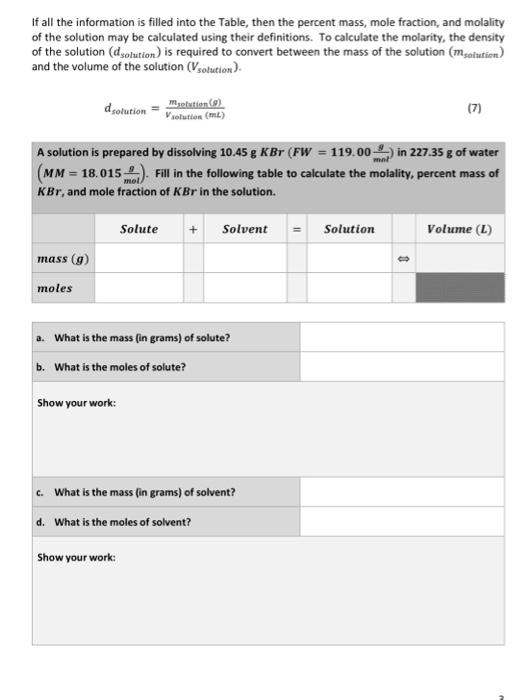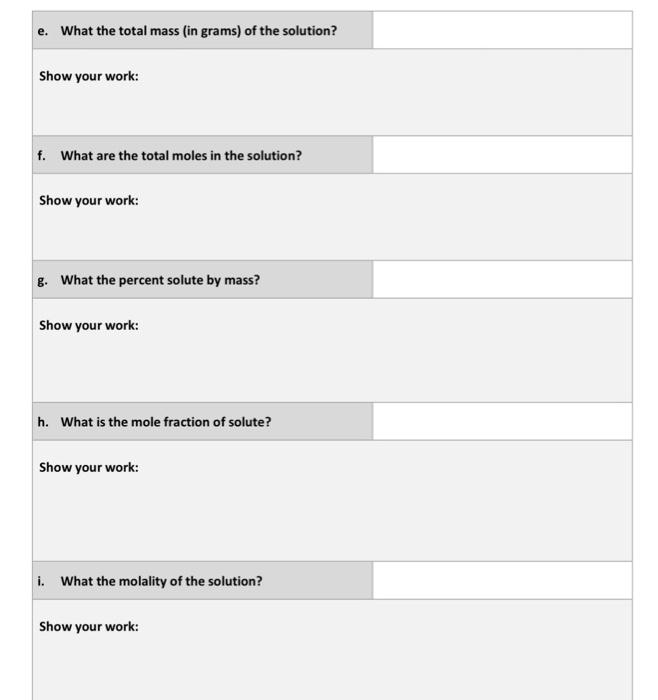Home / Expert Answers / Chemistry / if-all-the-information-is-filled-into-the-table-then-the-percent-mass-mole-fraction-and-molality-pa852

# (Solved): If all the information is filled into the Table, then the percent mass, mole fraction, and molality ...If all the information is filled into the Table, then the percent mass, mole fraction, and molality of the solution may be calculated using their definitions. To calculate the molarity, the density of the solution $$\left(d_{\text {solution }}\right)$$ is required to convert between the mass of the solution $$\left(m_{\text {solution }}\right)$$ and the volume of the solution $$\left(V_{\text {solution }}\right)$$. $d_{\text {solution }}=\frac{m_{\text {selution }}(g)}{V_{\text {selution }}(m L)}$ A solution is prepared by dissolving $$10.45 \mathrm{~g} \mathrm{KBr}\left(F W=119.00 \frac{\mathrm{g}}{\mathrm{mnl}}\right)$$ in $$227.35 \mathrm{~g}$$ of water $$\left(M M=18.015 \frac{g}{m o l}\right)$$. Fill in the following table to calculate the molality, percent mass of $$K B r$$, and mole fraction of $$K B r$$ in the solution. e. What the total mass (in grams) of the solution? Show your work: f. What are the total moles in the solution? Show your work: g. What the percent solute by mass? Show your work: h. What is the mole fraction of solute? Show your work: i. What the molality of the solution? Show your work:

We have an Answer from Expert